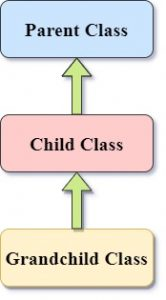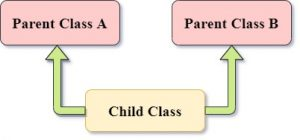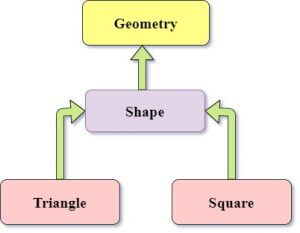Hi everyone, In this tutorial, we will learn about the concept of Inheritance in Python with some real-world examples.

## 1. What is Inheritance?

`Inheritance` is one of the four pillars of object-oriented programming and it allows us to reuse our code in a different way and new functionalities with little modification. Inheritance takes its name from the concept of inheriting some properties and behaviour from a parent class. For example, a child inherits traits from parents and have some of its own characteristics. In programming, the simplest form is when a child class is derived from other class which is a parent class.

Before moving further if you want to learn about classes and objects, refer to this tutorial and have a look at constructors in python in this tutorial.

## 2. Some Terminologies

Let’s look at some of the terms that are commonly used in inheritance.

### 2.1. Base/Parent Class

This is the class which is being inherited by another class.

``````class Base:
"""Data Members & Member Functions
of Base class
"""``````

### 2.2. Derived/child Class

This is the class which inherits another class or the base class. All the data members and methods of the base class are automatically invoked to be usable with the object of the derived class and this class can have some attributes of its own.

``````class Derived(Base):
"""Data Members & Member Functions
of Base class (No need to write again
unless overriding is to be done.)
"""
"""Data Members & Member Functions
of Derived class
"""``````

## 3. Types of Inheritance in Python

### 3.1. Single Inheritance

This is the simplest form of inheritance where a derived class inherits from one and only one base class as in a parent-child relationship.``````class Parent:
eyeColor = "black"
def info(self):
print("This is a parent class")

class Child(Parent):
def info(self):
print("This is a child class")

if __name__ == "__main__":
objC = Child()
objC.info()
print(objC.eyeColor)``````

Output:

``````This is a child class
black``````

We that the object of child class inherits the attribute eyeColor from the parent class and implemented its own method.

### 3.2. Multi-level Inheritance

This type of inheritance is present where a class indirectly inherits from a class as in a grandparent and grandchild relationship.  At least 3 classes are involved in this type of inheritance.``````class Parent:
eyeColor = "black"
def height(self):
print("Height is tall")
def bloodGroup(self):
print("O +ve")

class Child(Parent):
def bloodGroup(self):
print("B +ve")

class GrandChild(Child):
eyeColor="brown"

if __name__ == "__main__":
objGc = GrandChild()
objGc.bloodGroup()
objGc.height()
print("Eyecolor:",objGc.eyeColor)``````

Output:

``````B +ve
Height is tall
Eyecolor: brown``````

### 3.3. Multiple Inheritance

This type of inheritance is present where a class directly inherits from two or more (multiple) classes. If you have knowledge of JAVA programming, then you may have known that java does not support the concept of multiple inheritances directly. Like multi-level inheritance, in this type of inheritance also we need at least 3 classes.``````class ParentA:
eyeColor = "black"
def height(self):
print("Height is Average")

class ParentB:
eyeColor = "blue"
def height(self):
print("Height is Tall")
def bloodGroup(self):
print("Blood Group: O+")

class Child(ParentA,ParentB):
def __init__(self):
print("Created Object of Child class")

if __name__ == "__main__":
objC = Child()
objC.bloodGroup()
objC.height()
print("Eyecolor:",objC.eyeColor)``````

Output:

``````Created Object of Child class
Blood Group: O+
Height is Average
Eyecolor: black``````

In the above example, we see that child class inherits first from both the class and priority is given to the class from left to right order in the definition.

### 3.4. Hierarchical Inheritance

In Hierarchical inheritance, two or more (multiple) classes inherit from a single Base class. It is similar to a tree-like structure.``````class Area:
def __init__(self,shape):
print("Welcome to the world of {} !!".format(shape))

class Triangle(Area):
def __init__(self):
super().__init__('Triangles')
def area(self,h,b):
print("Area of Triangle: ",0.5*h*b)

class Square(Area):
def __init__(self):
super().__init__('Squares')
def area(self,s):
print("Area of Square: ",s*s)

if __name__ == "__main__":
sq = Square()
sq.area(6)
tri = Triangle()
tri.area(10,20)``````

Output:

``````Welcome to the world of Squares !!
Area of Square: 36
Welcome to the world of Triangles !!
Area of Triangle: 100.0``````

The `super()` keyword in the above is referring to the immediate parent class.

### 3.5. Hybrid Inheritance

This type of inheritance is made up of a combination of other types of inheritance. Let’s see an example of hybrid inheritance.``````class Geometry():
def __init__(self):
print("Welcome to Geometry !!")

class Shape(Geometry):
def __init__(self,shape):
super().__init__()
print("Created a {} !!".format(shape))

class Triangle(Shape):
def __init__(self):
super().__init__('Triangle')
def area(self,h,b):
print("Area of Triangle: ",0.5*h*b)

class Square(Shape):
def __init__(self):
super().__init__('Square')
def area(self,s):
print("Area of Square: ",s*s)

if __name__ == "__main__":
sq = Square()
sq.area(6)
tri = Triangle()
tri.area(10,20)``````

Output:

``````Welcome to Geometry !!
Created a Square !!
Area of Square: 36
Welcome to Geometry !!
Created a Triangle !!
Area of Triangle: 100.0``````

In the above example, we have combined the multi-level and hierarchical inheritance in a single program. Similarly,  we can create more combinations according to the requirement.

In this tutorial, we learned about Inheritance in Python and its types. If you have any doubt, feel free to ask in the comment section below.

## 4. Resources

Happy Learning 🙂

### About the Author: Kunal GuptaAspiring Data Scientist who loves Python Programming, Software Development and wants to Solve Real-world Problems.